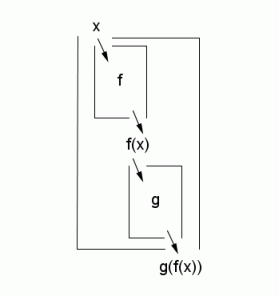﻿ Composition of Functions

#Composition of Functions

See this earlier lesson for a thorough introduction to function composition.

Make sure you can do all the exercises there before moving on to this page.

This current lesson adds information and exercises concerning the domain of a composite function.

DEFINITION function composition
The function $\,g\circ f\,$ (read as ‘$\,g\,$ circle $\,f\$’) is defined by: $$\cssId{s9}{(g\circ f)(x)} \cssId{s10}{:=} \cssId{s11}{g(f(x))}$$The function $\,g\circ f\$:
$\,f\,$ is ‘closest to’ the input, and acts first;
$\,g\,$ acts second

The domain of $\,g\circ f\,$ is the set of inputs $\,x\,$ with two properties:

• $\,f\,$ must know how to act on $\,x\,.$ That is, $\,x\in\text{dom}(f)\,.$
• $\,g\,$ must know how to act on $\,f(x)\,.$ That is, $\,f(x)\in\text{dom}(g)\,.$

Thus:

\begin{align} &\cssId{s18}{\text{dom}(g\circ f)}\cr &\quad \cssId{s19}{= \{x\ |\ x\in\text{dom}(f)\ \ \text{and}\ \ f(x)\in\text{dom}(g)\}} \end{align}

## Example

This example is contrived to give practice with the domain of a composite function.

PROBLEM:  Suppose that $\,f\,$ is the ‘add $\,3\,$’ function with a restricted domain:

$$\cssId{s27}{\text{dom}(f) = [0,2]}$$

Thus, $\,f\,$ only knows how to act on the numbers $\,0\le x\le 2\,.$

Suppose that $\,g\,$ is the ‘subtract $\,5\,$’ function with a restricted domain:

$$\cssId{s30}{\text{dom}(g) = [4,6]}$$

Find the domains of both $\,g\circ f\,$ and $\,f\circ g\,.$

SOLUTION:  We have:

$f(x) = x+3$  with  $\text{dom}(f) = [0,2]$

and

$g(x) = x-5\,$  with  $\text{dom}(g) = [4,6]$

Firstly, observe that the following sentences are equivalent:

$$\begin{gather} \cssId{s38}{f(x)\in \text{dom}(g)}\cr\cr \cssId{s39}{x+3\in [4,6]}\cr\cr \cssId{s40}{4\le x+3 \le 6}\cr\cr \cssId{s41}{1\le x\le 3}\cr\cr \cssId{s42}{x\in [1,3]} \end{gather}$$

Then,

\begin{align} &\cssId{s44}{\text{dom}(g\circ f)}\cr\cr &\quad\cssId{s45}{= \{x\ |\ x\in\text{dom}(f)\ \ \text{and}\ \ f(x)\in\text{dom}(g)\}}\cr\cr &\quad\cssId{s46}{= \{x\ |\ x\in [0,2]\ \ \text{and}\ \ x\in[1,3]\}}\cr\cr &\quad\cssId{s47}{= \{x\ |\ x\in ([0,2]\cap[1,3])\}}\cr\cr &\quad\cssId{s48}{= \{x\ |\ x\in [1,2]\}}\cr\cr &\quad\cssId{s49}{= [1,2]} \end{align}

Similarly (and a bit more compactly):

\begin{align} &\cssId{s51}{\text{dom}(f\circ g)}\cr\cr &\quad\cssId{s52}{= \{x\ |\ x\in\text{dom}(g)\ \ \text{and}\ \ g(x)\in\text{dom}(f)\}}\cr\cr &\quad\cssId{s53}{= \{x\ |\ x\in [4,6]\ \ \text{and}\ \ x-5\in[0,2]\}}\cr\cr &\quad\cssId{s54}{= \{x\ |\ x\in [4,6]\ \ \text{and}\ \ x\in[5,7]\}}\cr\cr &\quad\cssId{s55}{= [5,6]} \end{align}